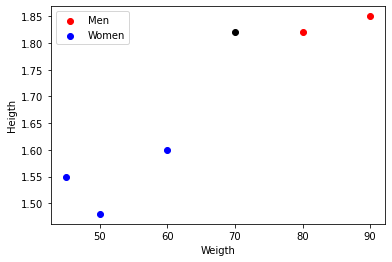Classification in Depth with Scikit-Learn

# Binary Classification: Programming Exercise

In this project you'll practice Binary classification using Decision Tree and KNN models. Binary classification is a common task in machine learning where the goal is to predict a binary outcome, such as true or false, yes or no, or positive or negative. By working on simple examples, you'll gain a better understanding of the concepts and techniques involved in binary classification.

## Project Activities

All our Data Science projects include bite-sized activities to test your knowledge and practice in an environment with constant feedback.

All our activities include solutions with explanations on how they work and why we chose them.

codevalidated

### Decision tree classification

For this implementation uses a decision tree classifier to predict the label of a fruit based on its color and weight. The data is stored in a pandas DataFrame and split into training and testing sets. The classifier is trained on the training data, and its accuracy is tested on the testing data.

Remember to encode the variable 'color' using, for example, 'get_dummies'. The models only understand numbers, not words or strings.

Split the dataset by using the function `train_test_split()`. You need to pass 3 parameters features, target, and test_set size. Split the dataset 30% test and 70% train and set using a `random state`=0. Store output of the split in `X_train`, `Y_train`, `X_test` and `Y_test`.

Then implement the decision tree using only `random_state=0`. Store the model in the variable `cf`, and finnaly estimate the accuracy of the model using the test dataset.

``````# Create the data for the fruit classifier
data = {'fruit': ['apple', 'banana', 'apple', 'banana', 'banana','apple','apple','apple'],
'color': ['red', 'yellow', 'green', 'yellow', 'yellow','green', 'green', 'red'],
'weight': [200, 100, 150, 90, 85,95,99,102],
'label': [0, 1, 0, 1, 1,0,0,0]}
df = pd.DataFrame(data)
``````

The results of the accuracy calculation should be stored in the variables `train_accuracy` and `test_accuracy` for the training and testing sets, respectively.

codevalidated

### K-NN classification model

We continue working with previous dataset, but for this task train a KNN classifier to predict the label of a fruit based on its color and weight.

Split the dataset by using the function `train_test_split()`. You need to pass 3 parameters features, target, and test_set size. Split the dataset 30% test and 70% train and set using a `random state`=0. Store output of the split in `X_train`, `Y_train`, `X_test` and `Y_test`. Remember that KNN is sensitive to the scale of the features, so you should use `StandardScaler` to standardize the features and store the results in the variables `X_train_scaler` and `X_test_scaler`.

Then implement the KNN using the argument for default. Store the model in the variable `knn`, and finnaly estimate the accuracy of the model using the test dataset.

``````# Create the data for the fruit classifier
data = {'fruit': ['apple', 'banana', 'apple', 'banana', 'banana','apple','apple','apple'],
'color': ['red', 'yellow', 'green', 'yellow', 'yellow','green', 'green', 'red'],
'weight': [200, 100, 150, 90, 85,95,99,102],
'label': [0, 1, 0, 1, 1,0,0,0]}
df = pd.DataFrame(data)
``````

The results of the accuracy calculation should be stored in the variables `train_accuracy` and `test_accuracy` for the training and testing sets, respectively.

multiplechoice

### Decision tree

What are the advantages of the decision tree?

multiplechoice

### Decision Tree Classifier

Choose the correct statement from below.

multiplechoice

### KNN

A student has a dataset with 500 data points that he want to use to train a KNN classifier. He trains 4 kNN classifiers (k={1,3,5,10}) using all the data points. Then you randomly select 300 data points from the 500, and classify them using each of the 4 classifiers.

Which classifier will come out as the best one?

multiplechoice

### KNN Classifier

Based on the following figure, identify the class of the black point if you train a K-NN algorithm with k=2.multiplechoice

### KNN and decision tree

Choose the correct statement from below.

multiplechoice

### KNN versus decision tree

For this task, we will use the following simulated dataset to train a decision tree and KNN models.

The data consists of information about 20 individuals, including their age, income, student status, and credit rating. The target variable, class, indicates whether an individual earns more or less than 50,000 a year (1 for more, 0 for less).

``````# Load the sample data
data = pd.DataFrame({'age': [23, 25, 22, 21, 24, 26, 20, 22, 19, 23, 25, 27, 21, 24, 22, 25, 26, 29, 31, 28],
'income': [50000, 60000, 55000, 65000, 65000, 70000, 45000, 62000, 48000, 50000, 67000, 72000, 49000, 55000, 65000, 62000, 72000, 75000, 85000, 90000],
'student': [0, 0, 1, 1, 0, 0, 1, 0, 1, 1, 0, 0, 1, 0, 1, 1, 0, 0, 1, 1],
'credit_rating': [620, 630, 600, 675, 635, 700, 625, 650, 575, 645, 725, 675, 550, 575, 600, 650, 720, 775, 800, 850],
'class': [0, 0, 0, 1, 1, 0, 1, 0, 1, 0, 0, 1, 1, 1, 1, 1, 1, 1, 1, 1]})
``````

The data is split into training and testing sets, and a decision tree and a KNN classifier were trained on the training data. The accuracy of the classifiers were evaluated on the testing data using the accuracy score and confusion matrix.

``````# Split the data into training and testing sets
X = data.drop(["class"], axis=1)
y = data["class"]
X_train, X_test, y_train, y_test = train_test_split(X, y, test_size=0.3, random_state=0)
``````

Your objective is to identify which models performed best in terms of the evaluation metric.Author

#### Verónica Barraza

This project is part of

## Classification in Depth with Scikit-Learn

Explore other projectsAnurag VermaAnurag VermaSantiago Basulto